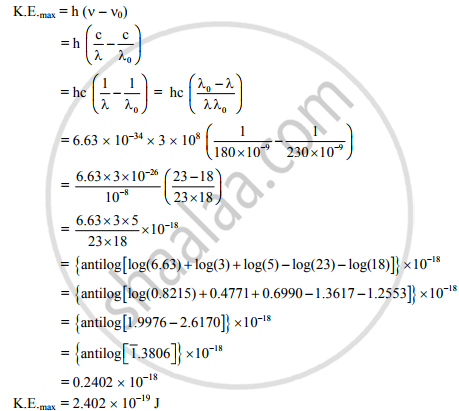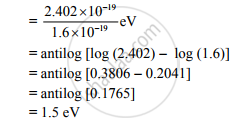HSC Science (Computer Science) 12th Board ExamMaharashtra State Board
Share

Determine the Maximum Kinetic Energy in Joule and in Ev of the Ejects Electron for the Metal Surface When It is Exposed to a Radiation of Wavelength 180 Nm. - HSC Science (Computer Science) 12th Board Exam - Physics

ConceptEinstein’s Equation - Particle Nature of Light

Question

The photoelectric threshold wavelength of a metal is 230 nm. Determine the maximum kinetic energy in joule and in eV of the ejects electron for the metal surface when it is exposed to a radiation of wavelength 180 nm.

[Planck’s constant : h = 6.63 * 10-34 Js, Velocity of light : C = 3 * 108 m/s.]

Solution

λ0 = 230 nm = 230 × 10−9m,

λ = 180 nm = 180 × 10−9m,
h = 6.63 × 10−34 J s, c = 3 × 108
m/s, K.E. (in joule and eV) = ?
Now,∴ The maximum kinetic energy of the ejected electron is 2.402 × 10−19 J.∴ The maximum kinetic energy of the ejected electron is 1.5 eV.

Is there an error in this question or solution?

APPEARS IN

2013-2014 (October) (with solutions)
Question 8.2 | 7.00 marks

Video TutorialsVIEW ALL 

Solution Determine the Maximum Kinetic Energy in Joule and in Ev of the Ejects Electron for the Metal Surface When It is Exposed to a Radiation of Wavelength 180 Nm. Concept: Einstein’s Equation - Particle Nature of Light.
S